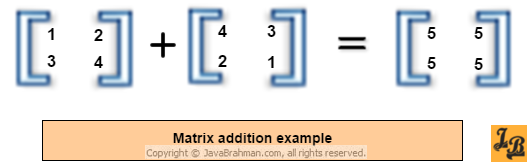Java program for matrix addition of dynamically sized matrices
Introduction This tutorial first explains the concept of matrix addition. It then provides the Java code for adding 2 matrices of any size or dynamically sized matrices. The program prints an error if the two matrices being added are not of the same size. How to add two matrices Matrix addition formula says -
Given two matrices, M and N, of same size, the sum of these matrices is obtained by adding the numbers - M ricj + N ricj to get the number in ricj position of the resulting matrix.
Where, r is a row with 0 < i < rows-1
c is a column with 0 < j < columns-1 .

The above formula in matrix form looks like this -An example of matrix addition would then look like this -Having understood how matrix addition is done, let us now see the java program for matrix addition, followed by the explanation of the code.
Java program for addition of 2 matrices
``````package com.javabrahman.generaljava;
public static void main(String args[]) {
int m1[][] = {{1, 2, 3},{1, 2, 3}};
int m2[][] = {{3, 4, 5},{3, 4, 5}};
if (sum != null) {
printMatrix(m1);System.out.println(" + ");printMatrix(m2);
System.out.println(" = ");printMatrix(sum);
}
}

/**
* Method to print a 2-D matrix row-wise
* @param matrix
*/
public static void printMatrix(int[][] matrix){
for (int i = 0; i < matrix.length; i++) {
for (int j = 0; j < matrix.length; j++) {
System.out.print(" "+matrix[i][j]);
}
System.out.println();
}
}

/**
* Method to add 2 matrices - m1 and m2 of any size
* Note - m1 & m2 should have same size
* @param m1
* @param m2
*/
public static int[][] addMatrices(int[][] m1, int[][] m2) {
int d1 = m1.length;
int d2 = m1.length;
int[][] sum = null;
if ((d1 != m2.length) || (d2 != m2.length)) {
System.out.println("Matrices not of same size. Cannot be added.");
} else {
sum = new int[d1][d2];
for (int i = 0; i < d1; i++) {
for (int j = 0; j < d2; j++) {
sum[i][j] = m1[i][j] + m2[i][j];
}
}
}
return sum;
}
}``````
OUTPUT of the above code
``` 1 2 3
1 2 3
+
3 4 5
3 4 5
=
4 6 8
4 6 8```
Explanation of the code
• The matrix addition logic is in the method named a`ddMatrices()`.
• `addMatrices()` method takes two 2-dimensional `int` arrays as input named `m1` and `m2`.
• It first checks if `m1` and `m2` are of same size i.e they have the same number of rows and columns.
• In case they are of different sizes then the method prints a message -"`Matrices not of same size. Cannot be added.`" and returns the `sum` as `null`.
• If `m1` and `m2` are of same size, then the method created two nested for-loops which move through each and every position of the matrices. As the loop iterates, the elements at the same row and column position in both the matrices are added and put in a third 2-dimensional array named '`sum`'. `sum` is our summed-up matrix which is returned at the end of the method.
• Note that `addMatrices()` is totally dynamic as it does not take the size of the matrices as input anywhere. It reads the size of the matrices dynamically using `m1.length `and `m1.length` into `d1` and `d2` variables and then uses these variables for looping through the matrices. The method is thus fully dynamic.
• The `printMatrix()` method simply prints the input matrix in a pretty format with matrix elements arranged in rows and columns.
• In the `main()` method two 2X2 matrices are initialized in variables `m1` and `m2` which are 2-dimensional `int` arrays. These matrices are printed along with their calculated sum as shown in the output above.
• To check the algorithm for 2-dimensional matrices of any other sizes, you can initialize them in `main()` and pass them to the `addMatrices()` method to get their sum as return value.
Summary In the above tutorial we first saw how matrix addition works and then saw the java program for implementing matrix addition along with detailed explanation of the code.